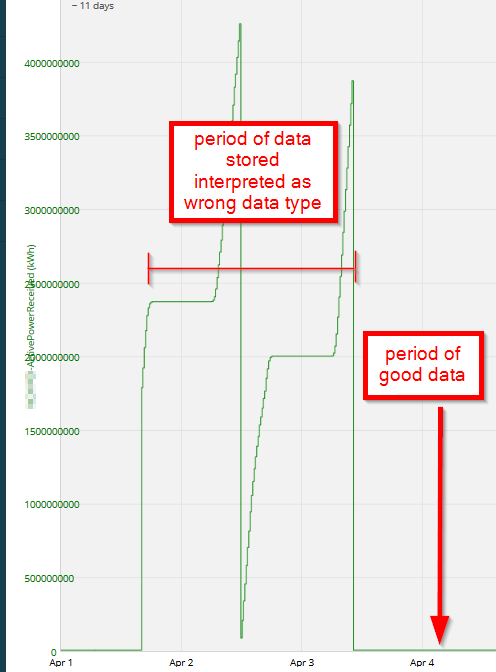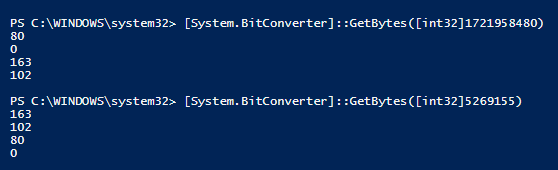# Prerequisites

While no special access is required to carry out the steps in this article, some level of familiarity with data types and PowerShell scripting is assumed.

# Problem

One or more tags contains data from a binary data source which was incorrectly cast to the wrong data type, and you want to salvage the data by extracting the binary data from Bazefield and converting it to the correct data type.

In this article, we will walk through an example where the tag for a 32-bit signed integer from a Modbus source was misconfigured. The Bazefield Modbus client interpreted the source data bytes as an unsigned 32-bit integer, and the resulting number was then stored in the Bazefield historian as either a 32 or 64 bit signed integer. As a result, a source data value of 5286458 would be incorrectly stored as 2855927888, throwing off trends and dependent calculations.Please note that the PowerShell script used in the article will likely need to be customized for each use case.

# Background

When binary data is received from a data source, the raw bytes are interpreted as the data type specified in the "IO Data Type" property of the corresponding tag. For example, if the data source sends a four-byte datum "00 50 66 8A", an int32 interpretation would be 5269130, a float32 interpretation would be 7.38x10^-39,  while an ASCII string interpretation would be (invalid)-P-f-(invalid). An online hex data type converter like this one can be used to interpret the raw bytes in different formats.

After the raw bytes are interpreted by Bazefield, they are cast to the data type specified in the tag's "Memory Data Type" before being archived in the Bazefield historian.

When data is exported from Bazefield via the Data Export tool or extracted by the Bazefield API, the historical data is retrieved as the string representation of the data that was archived as specified by the "Memory Data Type".

Therefore, to salvage the binary data which was incorrectly interpreted, we need to follow these same steps in reverse:

Data as string > data as "Memory Data Type" > data as bytes > reorder bytes to desired data type > interpret as correct "IO Data Type"

# Resolution

## Step 1: How important is this data to you, anyway?

This sort of data munging can be time-consuming. Depending on how important the data is, it may be acceptable to simply delete the incorrect data, or replace it with an interpolated value.

## Step 2: Obtain the raw data

The Bazefield Portal's "Data Export" tool is the easiest way to export raw data. Depending on the use case, the Bazefield API could also be an option.Next, import the data into a PowerShell session:

```\$inputFile = "PathToInputData.csv"
\$outputFile = "PathToOutputData.csv"
\$inputDataType1 = [int32]
\$inputDataType2 = [int64]

\$rawInputData = Import-Csv -Path \$inputFile -Delimiter ";"

\$columnHeaders = \$rawInputData | gm -MemberType NoteProperty | Select -ExpandProperty 'Name'

## Step 3: Cast the string data from the csv file to the "Memory Data Type"

In this example, the "Memory Data Type" was "Default", so we don't know a priori how the data was archived. However, since the source data was interpreted as an unsigned int32, the data would have been archived as either an int32 or int64 depending on its uint32 value. Therefore, in this case, before casting to the "Memory Data Type", it is necessary to check whether each data point should be interpreted as an int32 or an int64.

```#cast from string to inputDataTypes
foreach (\$entry in \$rawInputData)
{
# check if value was too big to be stored in int32, in which case try int64
if ((\$entry.\$tagName -as \$inputDataType2 -ne \$null) -and `
((\$entry.\$tagName -as \$inputDataType2 -gt \$inputDataType1::MaxValue) -or `
(\$entry.\$tagName -as \$inputDataType2 -lt \$inputDataType1::MinValue)))
{
(\$entry.\$tagName) = \$entry.\$tagName -as \$inputDataType2
}
# otherwise data was stored as int32
elseif (\$entry.\$tagName -as \$inputDataType1 -ne \$null)
{
(\$entry.\$tagName) = \$entry.\$tagName -as \$inputDataType1
}
}```

## Step 4: Convert from "Memory Data Type" to bytes

The .NET BitConverter class can be used to easily extract a byte array from each data point.

```#convert to byte arrays
\$inputBytes = New-Object 'Byte[][]' \$rawInputData.Count
for (\$i=0; \$i -lt \$inputBytes.Count; \$i++)
{
\$inputBytes[\$i] = [System.BitConverter]::GetBytes(\$rawInputData[\$i].\$tagName)
}```

## Step 5: Determine how the bytes should be reordered

Select an example data point, and manually test different byte orders until you find the correct reordering. In this example, we have an int32 value of 1721958480 which should actually have a value of 5269155.

1721958480 in bytes is "80 0 163 102"

5269155 in bytes is "163 102 80 0"Therefore, we need to reorder from indices 0, 1, 2, 3 to indices 2, 3, 0, 1.

64-bit integers can be similarly dissected and rearranged.

## Step 6: Reorder the bytes and convert to the destination data type

In this example, we check the length of each byte array to determine whether to treat it as an int32 or an int64.

```#output data
\$outputData = New-Object 'int32[]' \$rawInputData.Count
for (\$i=0; \$i -lt \$inputBytes.Count; \$i++)
{
# 4 byte swapping if data was stored as int32<span class="fr-marker" data-id="0" data-type="false" style="display: none; line-height: 0;"></span><span class="fr-marker" data-id="0" data-type="true" style="display: none; line-height: 0;"></span>
if (\$inputBytes[\$i].Count -eq 4)
{
\$swappedArray = @(\$inputBytes[\$i], \$inputBytes[\$i], \$inputBytes[\$i], \$inputBytes[\$i])
\$outputData[\$i] = [System.BitConverter]::ToInt32(\$swappedArray, 0)
}
# 8 byte swapping if data was stored as int64
elseif (\$inputBytes[\$i].Count -eq 8)
{
\$swappedArray = @(\$inputBytes[\$i], \$inputBytes[\$i], \$inputBytes[\$i], \$inputBytes[\$i], `
\$inputBytes[\$i], \$inputBytes[\$i], \$inputBytes[\$i], \$inputBytes[\$i])
\$outputData[\$i] = [System.BitConverter]::ToInt64(\$swappedArray, 0)
}
else
{
Write-Host "Skipping index \$i because data type with \$(\$inputBytes[\$i].Count) was not found"
}
}```

## Step 7: Output the data to a new csv file

Once the data has been converted and exported, you can use a number of options to import it back into Bazefield or use it for some other purpose.

```for (\$i=0; \$i -lt \$rawInputData.Count; \$i++)
{
Add-Member -InputObject \$rawInputData[\$i] -MemberType NoteProperty -Name "ConvertedData" -Value \$outputData[\$i]
}

\$rawInputData | Export-Csv -Path \$outputFile```

# More Details

In some cases, it may be necessary to conduct byte-swapping on many tags, which can be tedious using the procedure above. The "ByteSwapperMultipleTags.ps1" script attached to this article can be adapted for the purpose of conducting byte-swapping operations on a single data export that contains data for multiple tags.

# Product Environment and Version

Article last updated for Bazefield 8.0.16.3